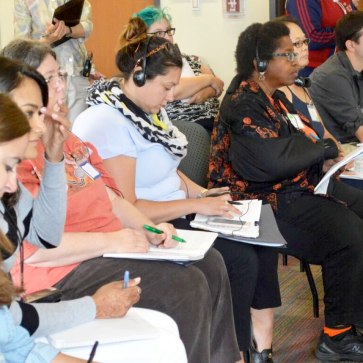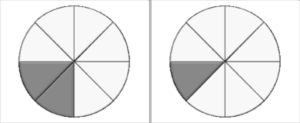# Conduct a Lesson Study Cycle on Fractions

This course supports your team to conduct a Lesson Study cycle on fractions. Your team should include at least one grade 3-5 teacher who will be teaching a fractions unit.

15-20 hours

##### MATERIALS:Module 2 | What Is Challenging About Fractions?

## What Makes Fractions So Difficult?

There’s something weird about fractions.  If the numerator, which is the top, is the same, the bigger the denominator the smaller the fraction.  - Jordan, Grade 5 student

Like Jordan, many students feel that “there’s something weird about fractions.”  The three tasks in this section shed some light on students’ challenges with fractions.

## Common Challenges with Fractions

From solving the three different fraction tasks and considering student solutions, summarize your team’s insights so far about the question:
• What is difficult for students about fractions?
The table “What’s Hard About Fraction Number Sense” summarizes six challenges in building fraction number sense, with examples of student understanding of each. Read each challenge, imagine what your students might find difficult, and click the challenge to see examples.

Discuss as a team:

• Anything in the table that is puzzling or particularly interesting to you
• Anything that helps you understand student responses to the tasks 1-3 that you examined

Record in Item 3 of the Teaching-Learning Plan any insights you would especially like to remember related to student thinking about fractions.

### What's Hard About Fraction Number Sense? (PDF)

 Challenge in Understanding Fractions Example of Student Difficulty or Understanding A Fraction is a Number A fraction represents an amount, not just pieces (such as 2 of 3 pieces of a pizza) or a situation (such as 2 of 3 shirts are red). When asked to put the fraction 2/3  on a number line, a student said “you can’t put it on a number line, because it’s two pieces out of three pieces, it’s not a number.” Or “ 2/3 is not a number, it’s two numbers.” [*] Fractions Can Be Greater than One May be difficult for students who have a strong image of a fraction as a piece of something. “You can’t have 6/5 because there’s only 5/5 in a whole.” Fractions Can Be Partitioned A whole can be divided into smaller and smaller equal parts. The same fractional quantity can be represented by different fractions. Difficulty seeing how to divide a whole into equal parts. Difficulty seeing that 1/2 is equal to 2/4, 3/6, 4/8, 5/10 and so on. What the Denominator Means Different units (such as 1/3  and 1/5) are different sizes. The more units a whole is partitioned into the smaller each one is. 1/n fits exactly n times into the whole. Students add 1/3 + 1/5 and get 2/8, without realizing they are adding two different things (thirds and fifths) sort of like adding apples and hammers. Students may think “1/5 is bigger than 1/4 because 5 is bigger than 4.” Difficulty seeing that 1/3 fits in the whole 3 times, 1/4 fits in the whole 4 times.  Has trouble seeing that 3/3, 4/4 etc. equal 1. Knowing What is the Whole Constructing the whole when given a fractional part. Keeping track of the whole. Difficulty making the whole when you give them a fractional part, e.g.: “This paper is 2/3; show me the whole.” Sees that the magnitude of a fraction depends on the magnitude of the whole (e.g., half of a small cookie is not the same as half of a large cookie) Confusion about whether the two drawings below together represent 3/8 of a pie or 3/16 of a pie.Fraction Size Understands that fraction size is determined by the (multiplicative) relationship between numerator and denominator - not just by the numerator, not just by the denominator, and not by the difference between numerator and denominator. Sees non-unit fraction as an accumulation of unit fractions. [A unit fraction has a numerator of 1; a non-unit fraction has a numerator other than 1.] May think 4/9 is bigger than 3/4 because 4 is bigger than 3 (comparing numerators), or 4/9 is bigger than 3/4 because 9 is bigger than 4 (comparing denominators), or 3/5 is the same size as 5/7 because the difference between the top and the bottom in both fractions is 2. Sees that equivalent fractions have the same multiplicative relationship between numerator and denominator. In 2/4, 4/8, 3/6, etc. denominator is two times numerator. Sees 5/8 is made up of five 1/8‘s or 5 times 1/8, that 9/8 is made up of 9 eighths or 9 times 1/8, etc.
[*] Kerslake, D. (1986). Fractions: Children’s strategies and errors. A report of the strategies and errors in Concepts in Secondary Mathematics and Science Project. Windsor, England: NFER-Nelson. Behr, M.J. & Post, T.R. (1992). Teaching rational numbers and decimal concepts. In T.R. Post (Ed.), Teaching mathematics in grades K-8, research-based methods (pp. 201-248). Boston: Allyn and Bacon.